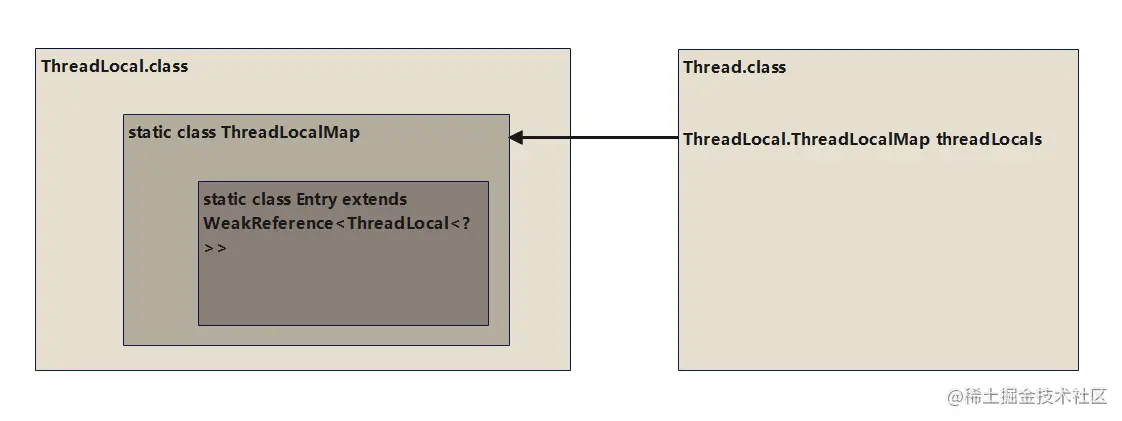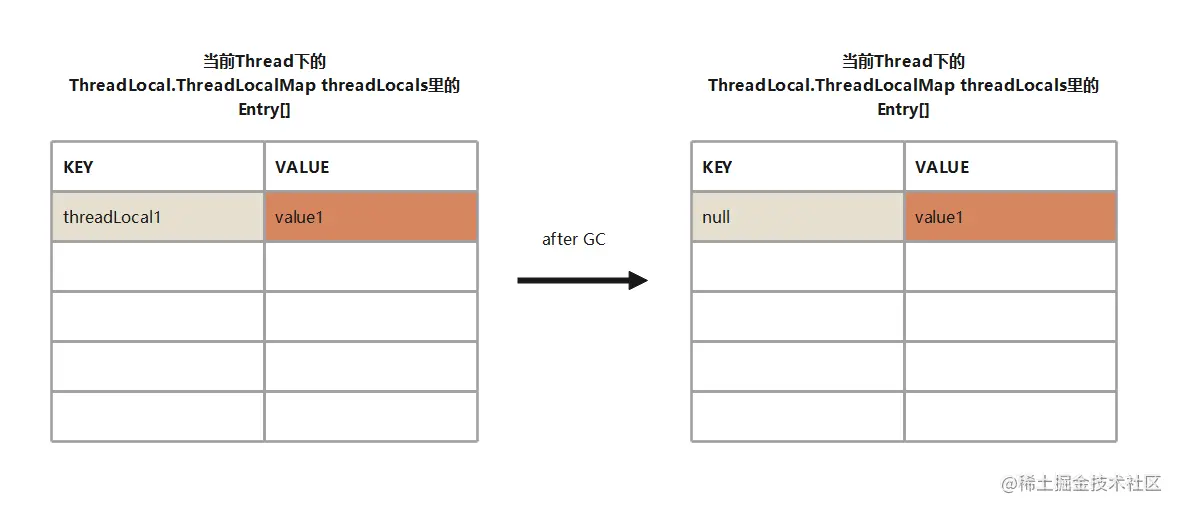#• 0码农老张 后端 2023-03-13

``````static ThreadLocal<Student> threadLocal = new ThreadLocal<Student>();

public static void main(String[] args) {
}

public void run() {
try {
TimeUnit.SECONDS.sleep(3);
} catch (InterruptedException e) {
e.printStackTrace();
}
}
}).start();

public void run() {
try {
TimeUnit.SECONDS.sleep(1);
} catch (InterruptedException e) {
e.printStackTrace();
}
}
}).start();
}

``````public void set(T value) {
// 获取当前线程
if (map != null)
map.set(this, value);
else
// 通过当前线程和调用set方法的入参对象去构造Map
createMap(t, value);
}

``````public T get() {
// 获取当前线程
if (map != null) {
if (e != null) {
@SuppressWarnings("unchecked")
// 获取Entry的value返回
T result = (T)e.value;
return result;
}
}
return setInitialValue();
}

``````ThreadLocal.ThreadLocalMap threadLocals = null

``````private void set(ThreadLocal<?> key, Object value) {
Entry[] tab = table;
// 取长度
int len = tab.length;
// 算下标
int i = key.threadLocalHashCode & (len-1);
for (Entry e = tab[i];
e != null;
e = tab[i = nextIndex(i, len)]) {
if (k == key) {
e.value = value;
return;
}
if (k == null) {
replaceStaleEntry(key, value, i);
return;
}
}
// 设置Entry
tab[i] = new Entry(key, value);
int sz = ++size;
if (!cleanSomeSlots(i, sz) && sz >= threshold)
rehash();
}

``````static class Entry extends WeakReference<ThreadLocal<?>> {
Object value;

super(k);
value = v;
}
}## WeakReference演示

``````public static void main(String[] args) {
wrTest();
}

private static void wrTest() {
WeakReference<Student> weakReference = new WeakReference<Student>(new Student("aaaa"));
System.out.println(weakReference.get());
System.gc();
System.out.println(weakReference.get());
}

``````Student@1b6d3586
null

## 内存泄漏``````public void remove() {
if (m != null){
m.remove(this);
}
}

Entry[] tab = table;
int len = tab.length;
int i = key.threadLocalHashCode & (len-1);
for (Entry e = tab[i]; e != null; e = tab[i = nextIndex(i, len)]) {
// 匹配key
if (e.get() == key) {
// 清除一个Entry元素
e.clear();
expungeStaleEntry(i);
return;
}
}
}

## 总结

Thread + Local = 线程 + 本地 = 线程本地变量 = 把某个对象放在了线程本地。